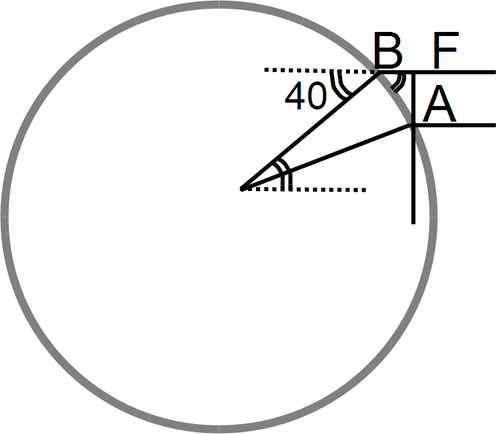20.3: A More Accurate Calculation

•• Contributed by CK12
• CK12

One reason an excessive value was obtained is that the Moon was assumed to be overhead at A or B. Actually, it is likely to be at some significant angle to the overhead direction, the “zenith" (see drawing).Figure $$\PageIndex{1}$$: Close up of the eclipse.

Then the section cut by the angle $$\alpha$$ from the circle of radius $$R$$ around E is not AB but AF (second drawing), which is smaller. Taking this into account reduces the distance.

We don't know where the Sun was during the 129 BC eclipse, but it must have been on the ecliptic (the words are obviously related!), which places it within 23.5° of the celestial equator, on either side. Assuming it was on the equator (that is, it passed overhead on the Earth's equator) and south of the reported observations (i.e. the eclipse occurred near noon) one can make a crude estimate of the correction, using simple trigonometry (see http://www.phy6.org/stargaze/Strig2.htm).

The Hellespont is around latitude of 40 degrees, and as the drawing shows, that is also the angle between the Moon's direction and the zenith. From the drawing:

$AF\,=\,AB\cos 40^∘\,=\,0.766AB$